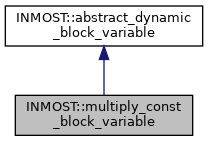INMOST A toolkit for distributed mathematical modeling
INMOST::multiply_const_block_variable Class Reference

This class represents multiplication of the matrix by the constant. More...

`#include <inmost_block_variable.h>`

Inheritance diagram for INMOST::multiply_const_block_variable:[legend]
Collaboration diagram for INMOST::multiply_const_block_variable:[legend]

## Public Member Functions

multiply_const_block_variable (const abstract_dynamic_block_variable &_ArgA, INMOST_DATA_REAL_TYPE val)
Constructor.

multiply_const_block_variable (const multiply_const_block_variable &b)
Copy constructor.

multiply_const_block_variableoperator= (multiply_const_block_variable const &b)
Assignment operator.

rMatrix Value (const Storage &e) const
Get value of variable expression on provided element e.

vMatrix Variable (const Storage &e) const
Get value with derivatives of variable expression on provided element e. More...

abstract_dynamic_block_variableCopy () const
Make a copy of this class, used to reproduce and store a tree of variable expressions.

## Detailed Description

This class represents multiplication of the matrix by the constant.

Definition at line 579 of file inmost_block_variable.h.

## ◆ Variable()

 vMatrix INMOST::multiply_const_block_variable::Variable ( const Storage & e ) const
inlinevirtual

Get value with derivatives of variable expression on provided element e.

This function collapses associated expression tree into multivar_expression.

Implements INMOST::abstract_dynamic_block_variable.

Definition at line 604 of file inmost_block_variable.h.

The documentation for this class was generated from the following file: